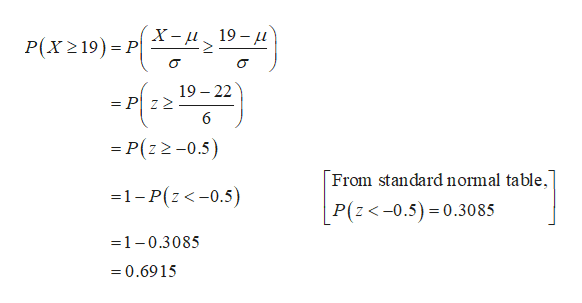# Suppose that the duration of a particular type of criminal trial is known to be normally distributed with a mean of 22 days and a standard deviation of 6 days. Let X be the number of days for a randomly selected trial. Round all answers to 4 decimal places where possible.a. What is the distribution of X? X ~ N(,)b. If one of the trials is randomly chosen, find the probability that it lasted at least 19 days. c. If one of the trials is randomly chosen, find the probability that it lasted between 21 and 24 days.

Question

Suppose that the duration of a particular type of criminal trial is known to be normally distributed with a mean of 22 days and a standard deviation of 6 days. Let X be the number of days for a randomly selected trial. Round all answers to 4 decimal places where possible.

a. What is the distribution of X? X ~ N(,)

b. If one of the trials is randomly chosen, find the probability that it lasted at least 19 days.

c. If one of the trials is randomly chosen, find the probability that it lasted between 21 and 24 days.

check_circleExpert Solution
Step 1

a)

The distribution of X ~ N(22,36) is and it is identified below:

Let X be the number of days for a randomly selected trial follows normal distribution with mean 22 days and a standard deviation of 6 days.

Step 2

b.

The probability that it lasted at least 19 days is 0.6915 a...help_outlineImage TranscriptioncloseX-и 19—д Р(x 219) - Р| 19 22 = P z2 6 = P(z 2-0.5) From standard normal table, 1-P(z<-0.5) P(z<-0.5) 0.3085 1-0.3085 =0.6915 fullscreen

### Want to see the full answer?

See Solution

#### Want to see this answer and more?

Solutions are written by subject experts who are available 24/7. Questions are typically answered within 1 hour*

See Solution
*Response times may vary by subject and question
Tagged in

### Other